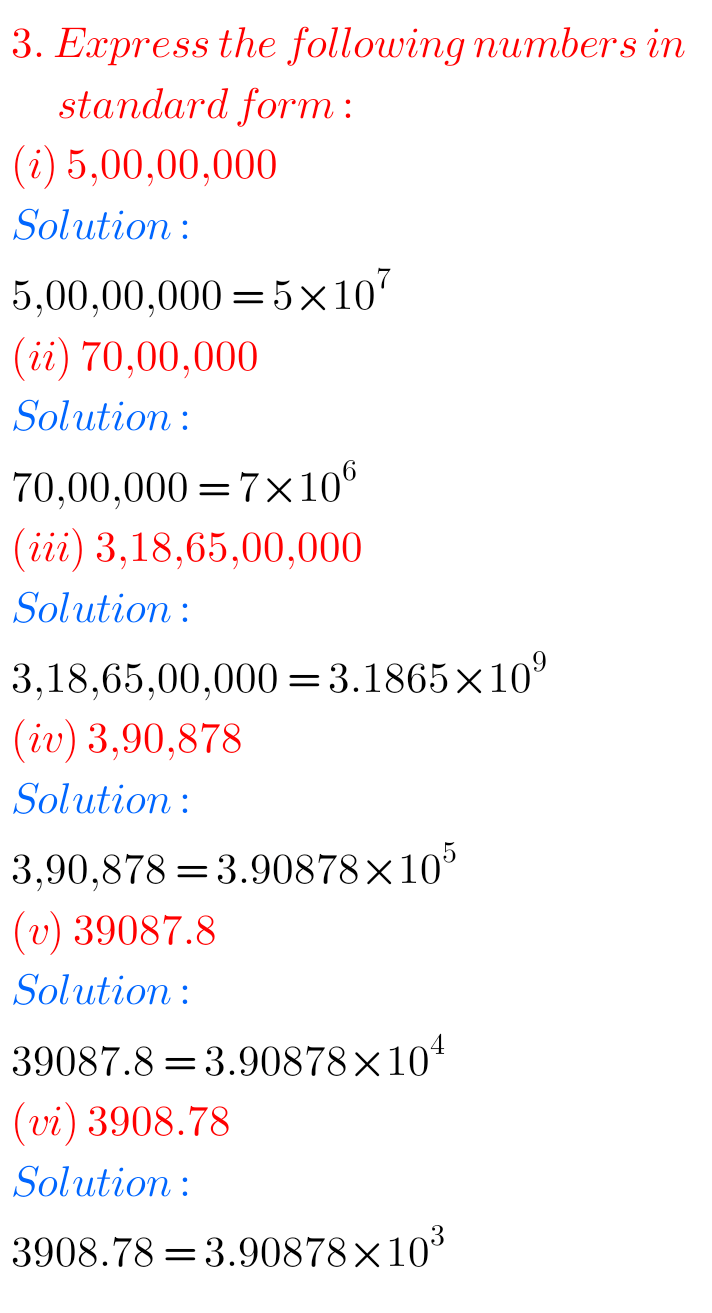# Uncategorized## NCERT Class 7 maths chapter 11 Exponents and Powers exercise 11.3 solutions

Exponents and Powers Exercise 11.3 solutions NCERT class 7 maths NCERT Mathematics class 7th chapter 11 Exponents and Powers Exercise 11.3 solutions are given. You should study the textbook lesson Exponents and Powers very well. You should also observe and practice all example problems and solutions are given in the textbook. Observe the given below …## Intermediate Maths Solutions for Trigonometric Equations Exercise 7(a)

Inter Maths Solutions for Trigonometric Equations Exercise 7(a) Inter Maths 1A Exercise 7(a) Trigonometric Equations solutions are given. These solutions are very easy to understand. Study the text book lesson very well. Observe the problems and solutions in the text book. Try them. Observe the solutions and try them in your own method. You can …